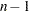Splines

Splines are curves, and they are usually required to be continuous and smooth. Splines are usually defined as piecewise polynomials of degree n with function values and firstderivatives that agree at the points where they join. The abscissa values of the join points are called knots. The term "spline" is also used for polynomials (splines with no knots) and piecewise polynomials with more than one discontinuous derivative. Splines with no knots are generally smoother than splines with knots, which are generally smoother than splines with multiple discontinuous derivatives. Splines with few knots are generally smoother than splines with many knots; however, increasing the number of knots usually increases the fit of the spline function to the data. Knots give the curve freedom to bend to follow the data more closely. See Smith (1979) for an excellent introduction to splines. There are many examples and detailed discussions of splines in Chapter 93, The TRANSREG Procedure. See the sections Linear and Nonlinear Regression Functions, Smoothing Splines, SPLINE and MSPLINE Transformations, Specifying the Number of Knots, SPLINE, BSPLINE, and PSPLINE Comparisons, Linear and Nonlinear Regression Functions, Simultaneously Fitting Two Regression Functions, and examples and Example 93.1.